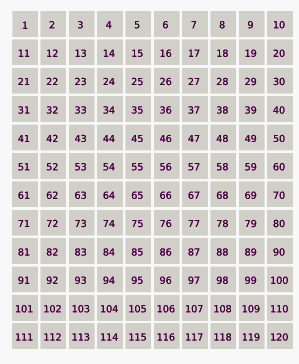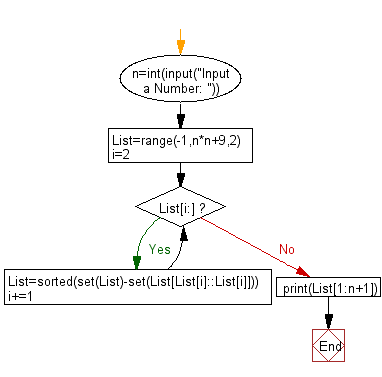﻿ Python Math: Print the first n Lucky Numbers - w3resource# Python Math: Print the first n Lucky Numbers

## Python Math: Exercise-17 with Solution

Write a Python program to print the first n Lucky Numbers.

Lucky numbers are defined via a sieve as follows.
Begin with a list of integers starting with 1 :
1, 2, 3, 4, 5, 6, 7, 8, 9, 10, 11, 12, 13, 14, 15, 16, 17, 18, 19, 20, 21, 22, 23, 24, 25, . . . .
Now eliminate every second number :
1, 3, 5, 7, 9, 11, 13, 15, 17, 19, 21, 23, 25, ...
The second remaining number is 3, so remove every 3rd number:
1, 3, 7, 9, 13, 15, 19, 21, 25, ...
The next remaining number is 7, so remove every 7th number:
1, 3, 7, 9, 13, 15, 21, 25, ...
Next, remove every 9th number and so on.
Finally, the resulting sequence is the lucky numbers.

Following animation demonstrating the lucky number sieve. The numbers in red are lucky numbers.Animation Credits: Celtic Minstrel

Sample Solution:-

Python Code:

``````n=int(input("Input a Number: "))
List=range(-1,n*n+9,2)
i=2
while List[i:]:List=sorted(set(List)-set(List[List[i]::List[i]]));i+=1
print(List[1:n+1])
```
```

Sample Output:

```Input a Number: 10
[1, 3, 7, 9, 13, 15, 21, 25, 31, 33]
```

Flowchart:## Visualize Python code execution:

The following tool visualize what the computer is doing step-by-step as it executes the said program:

Python Code Editor:

Have another way to solve this solution? Contribute your code (and comments) through Disqus.

What is the difficulty level of this exercise?

Test your Programming skills with w3resource's quiz.

﻿

```>>> students = [{'name': 'John', 'score': 98}, {'name': 'Mike', 'score': 94}, {'name': 'Jennifer', 'score': 99}]